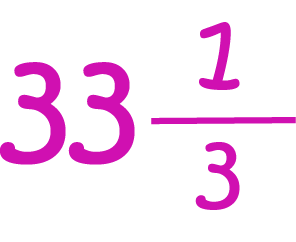# Definition of Mixed NumberA mixed number is a numeral that includes both a whole number and a fractional part. These are combined together to form one "mixed" number.

For example, $3\;\dfrac{3}{4}$ is a mixed number. Its whole number part is $3$, and its fractional part is $\dfrac{3}{4}$.

Another term for a mixed number is a mixed fraction.

### Description

The aim of this dictionary is to provide definitions to common mathematical terms. Students learn a new math skill every week at school, sometimes just before they start a new skill, if they want to look at what a specific term means, this is where this dictionary will become handy and a go-to guide for a student.

### Audience

Year 1 to Year 12 students

### Learning Objectives

Learn common math terms starting with letter M

Author: Subject Coach
You must be logged in as Student to ask a Question.